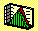2.1 OVERVIEW

Price changes in the capital markets are uncertain. And prices react constantly to events that occur around the world. As a result, the realized return from an investment decision usually differs from the return anticipated at the time of the investment. How investors deal with this uncertainty depends on their tolerance for risk and on the probability distribution of returns. The objective of this chapter is to provide quantitative measures of risk and expected return. These measures provide an important building block in the development of the capital asset pricing model.

In this development, we will take the description of uncertainty as being exogenous. This means that we will deal directly with the return distribution, and not question the factors that affect this distribution. For example, we do not consider how firms make decisions that affect the return distribution, such as production or marketing decisions. Instead, we take the distribution as given, and look at ways we can estimate it. There are two approaches to the estimation problem. The first we have labeled the financial analyst approach and the second the financial statistician approach.

This chapter explains the concepts of probability and probability distributions that are developed in topic 2.2, Probability. Topic 2.3, Population Statistics, covers the financial analyst approach. The pure statistical approach is covered in the topic 2.4, Sample Statistics. These two paths meet when we discuss the properties of estimators, such as unbiasedness and consistency.

Ultimately, this development feeds into the main topic of interest, topic 2.9, Portfolio Statistics. Here we discuss the important quantification of statistical building blocks required for the general investment problem: namely, how are the risk and return from a portfolio of securities measured? Modern portfolio theory assumes that the risk of a portfolio is measured by the standard deviation of portfolio returns. The expected return is measured by the mean or average return. We explain how to calculate the expected return and standard deviation of a portfolio, and present several examples of such calculations.

previous topic

next topic